# Peggy 2LE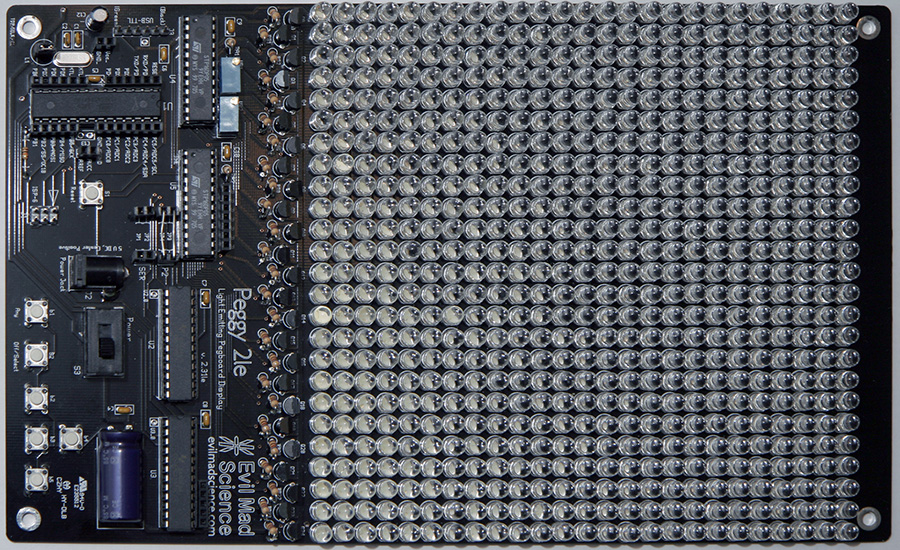Peggy 2LE is an open-source LED matrix "pegboard" display from Evil Mad Scientist Laboratories. It can be programmed from the Arduino development environment. You will need The Arduino Library for Peggy 2.0.

The web site says “Peggy 2LE does not have the breadboard-style prototyping area on board” which is true, but with some effort (solderless) prototyping is pretty easy. I added female headers everywhere I could. I did not solder in any of the jumpers - again headers make it possible to switch the serial hack on and off any time.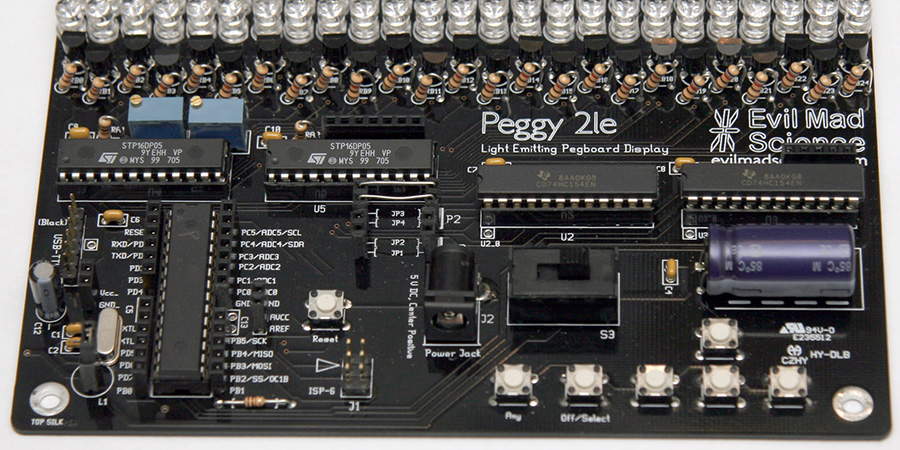## Accelerometer

My first application is to connect an accelerometer to the Peggy 2LE. I used the same setup that I used in my Arduino accelerometer project and because I used the female headers, it takes only seconds to make the necessary connections.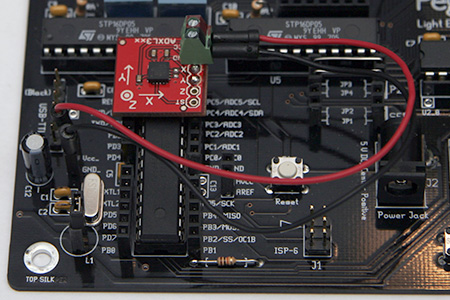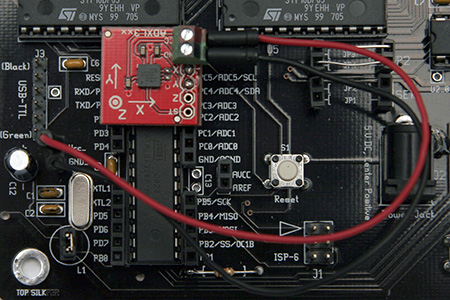The code is pretty simple:

```/**
* Peggy2Accel
*/

#include

#define ACCEL_X  5 // ADXL Y pin
#define ACCEL_Y  4 // ADXL X pin

Peggy2 frame1;

void setup()
{
frame1.HardwareInit();
}

void loop()
{
byte curr_x = constrain((analogRead(ACCEL_X) - 362) / 12, 0, 24);
byte curr_y = constrain((analogRead(ACCEL_Y) - 362) / 12, 0, 24);
curr_y = 24 - curr_y;
frame1.Clear();
frame1.SetPoint(curr_x, curr_y);
frame1.RefreshAll(1);
}
```

## Maze

My second application is a random maze generator. The 25×25 pixel would alternate between walls and maze. So each cell has always a wall in the NE, SE, SW, and NW corner and maybe a wall N, E, S, or W. Neighboring cells would share the wall / not wall. The first and last row / column would always be a wall. So the maze itself will only have 12×12 cells.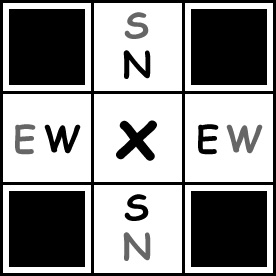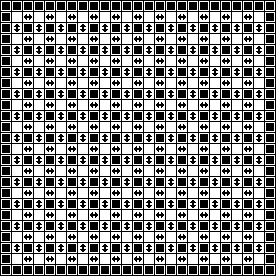I liked the simple method Depth-First Search. The problem is, that most implementations have a backtracking stack, which can become quite large (the number of the cells) on top of the maze data itself. Which means I would need 2 bytes for each cell to store the (x, y) location of the backtracking stack plus 1 byte for the wall information times 12×12 = 432 bytes. Plus the additional memory for the grayscale image is just too much. To preserve memory the trick is to store the backtracking information is the maze itself, like in MazeWorks. But even this is an overkill for this application - I only need the wall information and the backtracking information, which means everything fits in one byte per cell = 144 bytes total (which is great for the Peggy 2LE brain).

The Peggy 2LE code is here:

```/**
* Peggy2Maze
*/

#include <Peggy2Frame.h>

// brightness
#define WALL_0      0
#define WALL_1      2

// wall constants
#define WALL_N   0x10
#define WALL_E   0x20
#define WALL_S   0x40
#define WALL_W   0x80
#define WALL_ALL 0xF0

//                        N        E        S        W
byte MOVE_X[]   = {       0,       1,       0,      -1 };
byte MOVE_Y[]   = {      -1,       0,       1,       0 };

// AND clear mask for walls
byte WALL[]     = { ~WALL_N, ~WALL_E, ~WALL_S, ~WALL_W };

// dimensions of maze
#define DIM_X 12
#define DIM_Y 12

// size of maze
#define TOTAL DIM_X * DIM_Y

byte maze[TOTAL];

byte curr_x;
byte curr_y;

byte dir;

Peggy2Frame screen;

void setup()
{
}

void loop()
{
// choose random a cell and make it the current cell

curr_x = 11; //random(DIM_X);
curr_y = 11; //random(DIM_Y);
byte curr_i = curr_y * DIM_X + curr_x;

// create a maze of cells with all walls up, maze[] is a bit mask
// upper 4 bits are for what wall is up
// lowest 2 bits is step back direction
// 7   6   5   4   3   2   1   0
// W   S   E   N          D1  D0
for (byte i = 0; i < TOTAL; i++) maze[i] = WALL_ALL;

//------------------------------------------------------------
// GENERATE
//
byte visited = 1;
byte poss_dir[] = { 0, 0, 0, 0 };

while (visited < TOTAL) {
byte dir_cnt = 0;

// find all neighbors of current cell with all walls intact in order: N E S W
for (dir = 0; dir < 4; dir++) {
byte next_x = curr_x + MOVE_X[dir];
byte next_y = curr_y + MOVE_Y[dir];

//  check for valid next cell
if ((0 <= next_x) && (next_x < DIM_X) && (0 <= next_y) && (next_y < DIM_Y))
// check if previously visited
if (maze[next_y * DIM_X + next_x] == WALL_ALL)
// not visited, so add to possible next cells
poss_dir[dir_cnt++] = dir;
}

if (dir_cnt > 0) {
// current cell has one or more unvisited neighbors, so choose one at random
dir = poss_dir[random(dir_cnt)];

// clear current wall
maze[curr_i] &= WALL[dir];

// make next cell the current cell
curr_x += MOVE_X[dir];
curr_y += MOVE_Y[dir];
curr_i = curr_y * DIM_X + curr_x;

// find opposing dir by XOR 0x02, N 0x00 <-> S 0x02, E 0x01 <-> W 0x03
dir ^= 0x02;

// clear opposing wall
maze[curr_i] &= WALL[dir];

// step back direction
maze[curr_i] |= dir;

// increment count of visited cells
visited++;
}
else {
// reached dead end, backtrack by getting direction back from maze[]
dir = maze[curr_i] & 0x03;

// make prev cell the current cell
curr_x += MOVE_X[dir];
curr_y += MOVE_Y[dir];
curr_i = curr_y * DIM_X + curr_x;
}
}

//------------------------------------------------------------
// display
//
screen.Clear();
curr_i = 0;
byte x_ = 0;
byte y_ = 0;
for (byte y = 0; y < DIM_Y; y++) {
for (byte x = 0; x < DIM_X; x++) {
screen.SetPoint(x_++, y_, WALL_1);
screen.SetPoint(x_++, y_, (maze[curr_i++] & WALL_N) ? WALL_1 : WALL_0);
}
screen.SetPoint(x_++, y_, WALL_1);
x_ = 0;
y_++;

curr_i -= DIM_X;
for (byte x = 0; x < DIM_X; x++) {
screen.SetPoint(x_++, y_, (maze[curr_i++] & WALL_W) ? WALL_1 : WALL_0);
screen.SetPoint(x_++, y_, WALL_0);
}
screen.SetPoint(x_++, y_, WALL_1);
x_ = 0;
y_++;
}
for (byte x = 0; x < 2 * DIM_X + 1; x++)
screen.SetPoint(x_++, y_, WALL_1);

for (int frame = 0; frame < 1000; frame++) screen.Refresh();
}
```

This Java Script example demonstrates the algorithm. You can generate a new maze by reloading the page and you can see the source code by using "View Page Source" after you followed the link.